# Linear Regression in Machine Learning

In this page we will learn What is Linear Regression in Machine Learning?, Types of Linear Regression, Linear Regression Line, Gradient Descent, Model Performance, Assumptions of Linear Regression.

## What is Linear Regression in Machine Learning?

One of the most basic and widely used Machine Learning methods is linear regression. It's a statistical technique for performing predictive analysis. Sales, salary, age, product price, and other continuous/real or numeric variables are predicted using linear regression.

The linear regression algorithm reveals a linear relationship between a dependent (y) variable and one or more independent (y) variables, thus the name. Because linear regression reveals a linear relationship, it determines how the value of the dependent variable changes as the value of the independent variable changes.

The link between the variables is represented by a slanted straight line in the linear regression model. Consider the following illustration: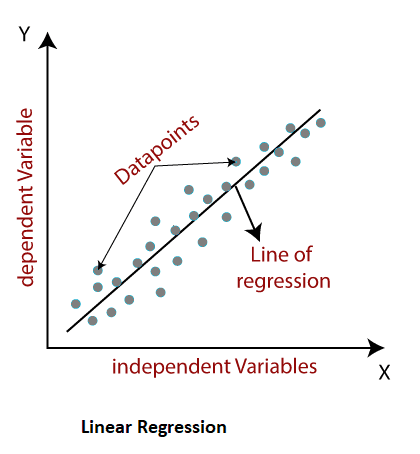Mathematically, we can represent a linear regression as:

y= a0+a1x+ ε

Here,
Y = Dependent Variable (Target Variable)
X = Independent Variable (predictor Variable)
a0= intercept of the line (Gives an additional degree of freedom)
a1 = Linear regression coefficient (scale factor to each input value).
ε = random error
The values for the x and y variables are training datasets for the representation of a Linear Regression model.

## Types of Linear Regression

Linear regression algorithms are further separated into two types:

• Simple Linear Regression: Simple Linear Regression is a Linear Regression algorithm that uses a single independent variable to predict the value of a numerical dependent variable.
• Multiple Linear Regression: It is a Linear Regression approach that uses more than one independent variable to predict the value of a numerical dependent variable.

## Linear Regression Line

A regression line is a straight line that depicts the relationship between the dependent and independent variables. There are two sorts of relationships that can be represented by a regression line:

• Positive Linear Relationship: A positive linear relationship exists when the dependent variable increases on the Y-axis while the independent variable increases on the X-axis.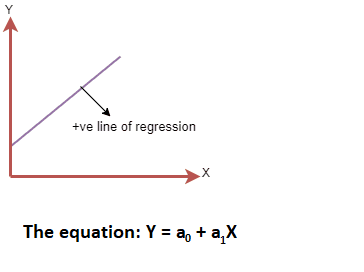• Negative Linear Relationship: A negative linear connection exists when the dependent variable declines on the Y-axis while the independent variable increases on the X-axis.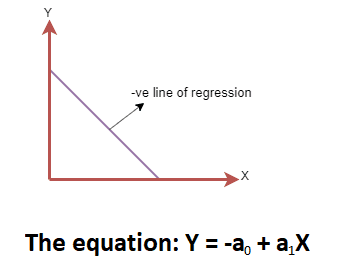## Finding the best fit line:

When using linear regression, our major goal is to identify the best fit line, which means that the difference between projected and actual values should be as little as possible. The line with the best fit will have the least amount of inaccuracy.

Different weights or coefficients of lines (a0, a1) produce different regression lines, thus we must determine the optimal values for a0 and a1 to obtain the best fit line, which we can do using the cost function.

Cost function-

• The cost function is used to estimate the values of the coefficient for the best fit line, and different values for weights or coefficient of lines (a0, a1) offer alternative lines of regression.
• The regression coefficients or weights are optimized using the cost function. It evaluates the performance of a linear regression model.
• The cost function can be used to determine the correctness of a mapping function that maps an input variable to an output variable. The Hypothesis function is another name for this mapping function.

We utilize the Mean Squared Error (MSE) cost function for Linear Regression, which is the average of squared errors between predicted and actual values. It is possible to write it.

For the above linear equation, MSE can be calculated as: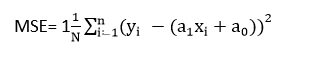Where,
N=Total number of observation

Yi = Actual value

(a1xi+a0)= Predicted value.

Residuals: Residuals are the differences between the actual value and the projected value. The residual will be high if the observed points are distant from the regression line, and the cost function will be high if the observed points are far from the regression line. If the scatter points are close to the regression line, the residual and hence the cost function will be tiny.

• By calculating the gradient of the cost function, gradient descent is utilized to minimize the MSE.
• Gradient descent is a technique for updating line coefficients by lowering the cost function in a regression model.
• It is accomplished by selecting a random set of coefficient values and then iteratively updating the values to arrive at the lowest cost function.

## Model Performance:

The Goodness of Fit is a measure of how well a regression line fits a collection of data. Optimization is the process of selecting the optimal model from a set of options. It can be accomplished using the following method:

1. R-squared method:

• The goodness of fit is determined using R-squared, a statistical method.
• On a scale of 0-100 percent, it assesses the strength of the link between the dependent and independent variables.
• A high R-square value indicates that there is a little difference between predicted and actual values, indicating a good model.
• For multiple regression, it's also known as a coefficient of determination or a coefficient of multiple determination.
• It can be calculated using the formula below: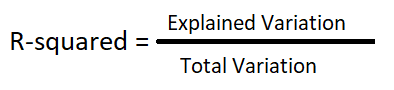## Assumptions of Linear Regression

The following are some key assumptions in linear regression. These are some formal checks that should be performed while developing a Linear Regression model to verify that the best possible result is obtained from the dataset.

• Linear relationship between the features and target: Linear regression presupposes that the dependent and independent variables have a linear relationship.
• Small or no multicollinearity between the features: The term "multicollinearity" refers to a high degree of correlation between the independent variables. Due to multicollinearity, determining the true link between predictors and target variables may be challenging. Or, to put it another way, determining which predictor variable affects the target variable and which does not is challenging. As a result, the model implies that the features or independent variables have little or no multicollinearity.
• Homoscedasticity Assumption: Homoscedasticity occurs when the error term is the same for all values of independent variables. In a scatter plot with homoscedasticity, there should be no discernible pattern distribution of data.
• Normal distribution of error terms: According to linear regression. When error terms are not normally distributed, confidence intervals become either too wide or too narrow, making determining coefficients problematic.

The q-q plot can be used to verify this. If the figure depicts a straight line with no deviations, the error is regularly distributed.
• No autocorrelations: In error terms, the linear regression model assumes no autocorrelation. If there is any correlation in the error term, the model's accuracy will be substantially reduced. If there is a reliance between residual errors, autocorrelation is likely to arise.